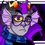# How to solve "nested" problems

Something that I see a lot at math competitions or on Brilliant is a problem asking to find the value of $\sqrt{x+\sqrt{x+\sqrt{x+\ldots}}}$ for $x=$ some value. I'm going to talk about how to solve that "nested radical" as well as "nested" fractions.

First I'm going to talk about how to solve a problem such as finding the value of $\sqrt{6+\sqrt{6+\sqrt{6+\ldots}}}.$ Let $k=\sqrt{6+\sqrt{6+\sqrt{6+\ldots}}}.$ You may notice that you are adding $k$ to $6$ and taking the square root of this. I'll demonstrate this with some parentheses. $\sqrt{6+\sqrt{6+\sqrt{6+\ldots}}}=\sqrt{6+\left(\sqrt{6+\sqrt{6+\ldots}}\right)}=\sqrt{6+\sqrt{\left(6+\sqrt{6+\ldots}\right)}}=\ldots$ For simplicity, we're just going to use $\sqrt{6+\sqrt{6+\sqrt{6+\ldots}}}=\sqrt{6+\left(\sqrt{6+\sqrt{6+\ldots}}\right)}.$ You may notice that this is the same thing as saying that $k=\sqrt{6+k}.$ Let's try solving that. \begin{aligned} k&=\sqrt{6+k}\\ k^2&=6+k\\ k^2-k-6&=0\\ (k-3)(k+2)&=0\\ k=3\text{ or }k=-2\\ \end{aligned} We can eliminate the $k=-2$ solution because the nested radical obviously is non-negative. Thus, we can say that $\sqrt{6+\sqrt{6+\sqrt{6+\ldots}}}=\boxed{3}.$

Now we can use a similar line of logic to find a general form for $\sqrt{x+\sqrt{x+\sqrt{x+\ldots}}}.$ Let that equal $y.$ We will make use of the same procedure, but using the quadratic formula to solve for $y.$ \begin{aligned} y&=\sqrt{x+\sqrt{x+\sqrt{x+\ldots}}}\\ y&=\sqrt{x+y}\\ y^2&=x+y\\ y^2-y-x&=0\\ y&=\dfrac{1\pm\sqrt{1+4x}}{2}\\ \end{aligned} Since we are dealing with real numbers, the value under the radical cannot be negative (except for $x=0$.) Thus, we can say that $\sqrt{x+\sqrt{x+\sqrt{x+\ldots}}}=\dfrac{1+\sqrt{1+4x}}{2}.$

Now let's use the same logic to derive a formula for nested fractions of the form $\dfrac{1}{a+\frac{1}{a+\ddots}}$ as well as continued fractions where the numerator is not necessarily $1.$

First, the obligatory demonstration of such a fraction. \begin{aligned} \dfrac{1}{2+\frac{1}{2+\ddots}}&=\dfrac{1}{2+\left(\frac{1}{2+\ddots}\right)}\\ k&=\dfrac{1}{2+k}\\ k^2+2k&=1\\ k^2+2k-1&=0\\ k&=\dfrac{-2\pm\sqrt{4+4}}{2}\\ k&=-1\pm\sqrt{2} \end{aligned} Again, we can throw out the negative solution and say that $\dfrac{1}{2+\frac{1}{2+\ddots}}=-1+\sqrt{2}.$

Moving on, we can derive a general formula for finding $\dfrac{c}{x+\frac{c}{x+\ddots}},$ allowing this expression to be equal to $y.$

\begin{aligned} y&=\dfrac{c}{x+\frac{c}{x+\ddots}}\\ y&=\dfrac{c}{x+y}\\ y^2+xy&=c\\ y^2+xy-c&=0\\ y&=\dfrac{-x+\sqrt{x^2+4c}}{2} \end{aligned}

So a summary of what we have proved here:

• $\sqrt{x+\sqrt{x+\sqrt{x+\ldots}}}=\dfrac{1+\sqrt{1+4x}}{2}$
• $\dfrac{c}{x+\frac{c}{x+\ddots}}=\dfrac{-x+\sqrt{x^2+4c}}{2}$

If you want some practice problems, here's two.

1. Find a closed form for $x+\dfrac{1}{2x+\frac{1}{2x+\ddots}}.$

2. This calculus problem

3. Find all values of $n$ for which $\sqrt{n+\sqrt{n+\sqrt{n+\ldots}}}$ is an integer.

I hope this helps you out! Please feel free to share what you think in the comments. Thank you very much!Note by Trevor B.
5 years, 9 months ago

This discussion board is a place to discuss our Daily Challenges and the math and science related to those challenges. Explanations are more than just a solution — they should explain the steps and thinking strategies that you used to obtain the solution. Comments should further the discussion of math and science.

When posting on Brilliant:

• Use the emojis to react to an explanation, whether you're congratulating a job well done , or just really confused .
• Ask specific questions about the challenge or the steps in somebody's explanation. Well-posed questions can add a lot to the discussion, but posting "I don't understand!" doesn't help anyone.
• Try to contribute something new to the discussion, whether it is an extension, generalization or other idea related to the challenge.

MarkdownAppears as
*italics* or _italics_ italics
**bold** or __bold__ bold
- bulleted- list
• bulleted
• list
1. numbered2. list
1. numbered
2. list
Note: you must add a full line of space before and after lists for them to show up correctly
paragraph 1paragraph 2

paragraph 1

paragraph 2

[example link](https://brilliant.org)example link
> This is a quote
This is a quote
    # I indented these lines
# 4 spaces, and now they show
# up as a code block.

print "hello world"
# I indented these lines
# 4 spaces, and now they show
# up as a code block.

print "hello world"
MathAppears as
Remember to wrap math in $$ ... $$ or $ ... $ to ensure proper formatting.
2 \times 3 $2 \times 3$
2^{34} $2^{34}$
a_{i-1} $a_{i-1}$
\frac{2}{3} $\frac{2}{3}$
\sqrt{2} $\sqrt{2}$
\sum_{i=1}^3 $\sum_{i=1}^3$
\sin \theta $\sin \theta$
\boxed{123} $\boxed{123}$

Sort by:

$\displaystyle \sqrt{x + \sqrt{x - \sqrt{x + \ldots }}} = \dfrac{1 + \sqrt{4x -3}}{2}$

$\displaystyle \sqrt{x - \sqrt{x + \sqrt{x - \ldots }}} = \dfrac{-1 + \sqrt{4x - 3}}{2}$

- 5 years, 9 months ago

How does one obtain these formulaes ?

- 1 year, 2 months ago

If you want to practice some problems on Nested Radicals ,
you may refer to my set Nested Radicals

- 5 years, 9 months ago

Thanks dude, I'll keep your tips it in mind while solving such questions $\ddot\smile$

- 5 years, 9 months ago

This is excellent. Thanks Trevor.

Staff - 5 years, 9 months ago

3.Let $\sqrt{n+\sqrt{n+\sqrt{\cdots}}}=x$.Then we can write it as $\sqrt{n+x}=x$ as you have just shown.Now solving: $n+x=x^2\\ x^2-x-n=0\\ x=\frac{-1\pm\sqrt{(-1)^2-4(1)(-n)}}{2(1)}\\ x=\frac{-1\pm\sqrt{4n+1}}{2}$ As the nested radical is positive,so we discard the negative square root.And we are left with: $\boxed{x=\frac{-1+\sqrt{4n+1}}{2}}$ By observation we conclude that $4n+1$ must be a perfect square odd number.That's as far as I got.Perhaps someone could complete this.

- 5 years, 9 months ago

My workings are similar to yours. I think your application of the quadratic equation is wrong though. If I am mistaken please correct me!

x = [1 +sqrt(1+4n)] / 2

x is an integer when 1 + 4n is a perfect square (and positive). 1 + 4n is an odd perfect square, therefore its square root is an odd integer.

Let (y + 1)^2 = 1 + 4n y^2 + 2y + 1 = 1+ 4n 4n = y^2 + 2y n = [y^2 + 2y] / 4 = y^2/4 + y/2 y is an even integer.

Thus n is an integer, whereby for all even integers y: n = (y^2)/4 + y/2

- 3 years, 7 months ago

Thank you :)

- 5 years, 9 months ago